# 22.12: Some Conjugated Cyclic Polyenes

$$\newcommand{\vecs}{\overset { \rightharpoonup} {\mathbf{#1}} }$$ $$\newcommand{\vecd}{\overset{-\!-\!\rightharpoonup}{\vphantom{a}\smash {#1}}}$$$$\newcommand{\id}{\mathrm{id}}$$ $$\newcommand{\Span}{\mathrm{span}}$$ $$\newcommand{\kernel}{\mathrm{null}\,}$$ $$\newcommand{\range}{\mathrm{range}\,}$$ $$\newcommand{\RealPart}{\mathrm{Re}}$$ $$\newcommand{\ImaginaryPart}{\mathrm{Im}}$$ $$\newcommand{\Argument}{\mathrm{Arg}}$$ $$\newcommand{\norm}{\| #1 \|}$$ $$\newcommand{\inner}{\langle #1, #2 \rangle}$$ $$\newcommand{\Span}{\mathrm{span}}$$ $$\newcommand{\id}{\mathrm{id}}$$ $$\newcommand{\Span}{\mathrm{span}}$$ $$\newcommand{\kernel}{\mathrm{null}\,}$$ $$\newcommand{\range}{\mathrm{range}\,}$$ $$\newcommand{\RealPart}{\mathrm{Re}}$$ $$\newcommand{\ImaginaryPart}{\mathrm{Im}}$$ $$\newcommand{\Argument}{\mathrm{Arg}}$$ $$\newcommand{\norm}{\| #1 \|}$$ $$\newcommand{\inner}{\langle #1, #2 \rangle}$$ $$\newcommand{\Span}{\mathrm{span}}$$$$\newcommand{\AA}{\unicode[.8,0]{x212B}}$$

## Azulene

There are several compounds that possess some measure of aromatic character typical of benzene, but do not possess a benzenoid ring. Appropriately, they have $$\left( 4n + 2 \right)$$ $$\pi$$ electrons and are classified as nonbenzenoid aromatic compounds (Section 21-9). An example is azulene, which is isomeric with naphthalene and has a five- and a seven-membered ring fused through adjacent carbons:As the name implies, it is deep blue. It is less stable than naphthalene, to which it isomerizes quantitatively on heating above $$350^\text{o}$$ in the absence of air:Azulene has a significant polarity, with the five-membered ring negative and the seven-membered ring positive. The structure can be represented as a hybrid of neutral and ionic structures: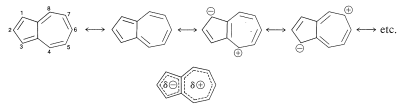The polarization that has the five-membered ring negative and the seven-membered ring positive corresponds to ionic structures that have six (i.e., $$4n + 2$$) electrons in both the five- and seven-membered rings (Section 21-9B).

In keeping with its aromatic character and unsymmetrical charge distribution, azulene undergoes certain typical electrophilic substitution reactions at the 1 and 3 positions. Thus Friedel-Crafts acylation leads to a mixture of 1-ethanoylazulene and 1,3-diethanoylazulene: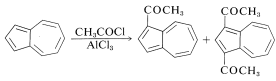Furthermore, in the presence of strong acids the 1 position is protonated to give a derivative of the relatively stable cycloheptatrienyl (tropylium) ion (Section 21-9B):## Cyclooctatetraene

1,3,5,7-Cyclooctatetraene (or simply cyclooctatetraene) is a bright-yellow, nonplanar, nonaromatic compound (Section 21-9A). Apparently the resonance energy of a planar structure is insufficient to overcome the unfavorable angle strain of a planar structure would have with its $$\ce{C-C-C}$$ bond angles of $$135^\text{o}$$. Cyclooctatetraene normally assumes a "tub" structure with alternating single and double bonds:There is, however, nmr evidence that indicates that the tub form is in rapid equilibrium with a very small amount of the planar form at room temperature. There is about a $$15$$-$$\text{kcal mol}^{-1}$$ energy difference between the two forms. The dication, $$\ce{C_8H_8^{2+}}$$, and the dianion of cyclooctatetraene, $$\ce{C_8H_8^{2-}}$$, which have $$\left( 4n + 2 \right)$$ $$\pi$$ electrons, appear to exist in planar conformation.

Cyclooctatetraene can be prepared readily by polymerization of ethyne in the presence of nickel cyanide: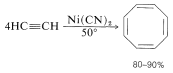It could be manufactured on a large scale, but no large-scale commercial uses of the substance have yet been developed.

The chemistry of cyclooctatetraene is interesting and unusual. Particularly noteworthy is the way in which it undergoes addition reactions to form products that appear to be derived from the bicyclic isomer, bicyclo[4.2.0]2,4,7-octatriene, $$8$$. In fact, there is an electrocyclic equilibrium between cyclooctatetraene and $$8$$ (Section 21-10D) and, although the position of equilibrium lies far on the side of cyclooctatetraene, $$8$$ is more reactive and leads to the observed addition products: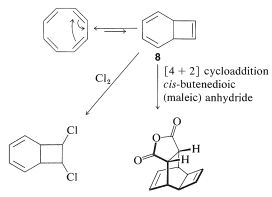Treatment of the bridged dichloride with strong bases causes elimination of hydrogen chloride and formation of chlorocyclooctatetraene: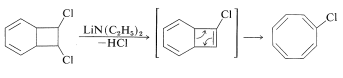The diverse ways in which cyclooctatetraene can react with a give reagent under different conditions is well illustrated by the variety of products obtained with mercuric ethanoate in ethanoic acid, methanol, and water:Efforts to prepare "pentalene", a bridged analog of cyclooctatetraene, have not been very successful so far. A substance that appears to be a methylpentalene has been characterized at $$-180^\text{o}$$ by its spectral properties. On warming to $$-105^\text{o}$$ it forms a dimer.## Annulenes

Cyclooctatetraene is nonplanar. One reason is that the angle strain is severe in the planar form. Is it possible that larger-ring conjugated polyalkenes may have strainless planar structures? Models show that a strainless structure can be achieved with two or more of the double bonds only in trans configurations, and then only with a large enough ring that the "inside" hydrogens do not interfere with one another.

In discussing compounds of this type, it will be convenient to use the name [n]annulene to designate the simple conjugated cyclic polyalkenes, with n referring to the number of carbons in the ring - benzene being annulene. The simplest conjugated cyclic polyolefin that could have a strainless planar ring containing trans double bonds, except for interferences between the inside hydrogens, is annulene:Inside-hydrogen interferences are likely to be of some importancee in all annulenes up to annulene. Many annulenes have been synthesized by F. Sondheimer.

We have mentioned already (Section 22-3C) the large differences in nmr chemical shifts between the inside and outside hydrogens of annulene - a substance which with 18 $$\pi$$ electrions should be aromatic by the $$4n + 2$$ rule. These differences are observed only at low temperatures. The proton nmr spectrum of annulene at room temperature is a single resonance, which indicates that the inside $$\left( \ce{H}_a \right)$$ and outside $$\left( \ce{H}_b \right)$$ hydrogens are equilibrating rapidly. This can take place only if cis-trans interconversion occurs about the double bonds (marked $$c$$ and $$t$$):At low temperatures, this equilibrium is slow enough that separate groups of resonances for the inside and outside hydrogens can be discerned in an nmr experiment (see Sections 9-10C and 27-2).

A theoretical prediction that has been borne out by experiment is that an annulene with $$4n$$ $$\pi$$ electrons should have a paramagnetic circulation of electrons - that is, opposite in direction to that shown in Figure 22-4 for benzene. For example, annulene, which has $$4n$$ electrons, is not very stable and exists as a very rapidly interconverting mixture of two configurational isomers:At very low temperatures $$\left( -155^\text{o} \right)$$, the proton nmr spectrum shows the inner hydrogens at $$\delta 12.9$$-$$10.5$$ and the outer hydrogens at $$\delta 5.7$$-$$6.4$$, which is in exactly the opposite order to the shifts with annulene and the other known $$\left[ 4n + 2 \right]$$ $$\pi$$-electron annulenes.

The annulenes generally are not stable compounds, but the $$\left[ 4n + 2 \right]$$annulenes clearly show typical aromatic reactions. For instance annulene has been converted to the nitro, ethanoyl, bromo, and carbaldehyde derivatives by electrophilic substitution reactions.